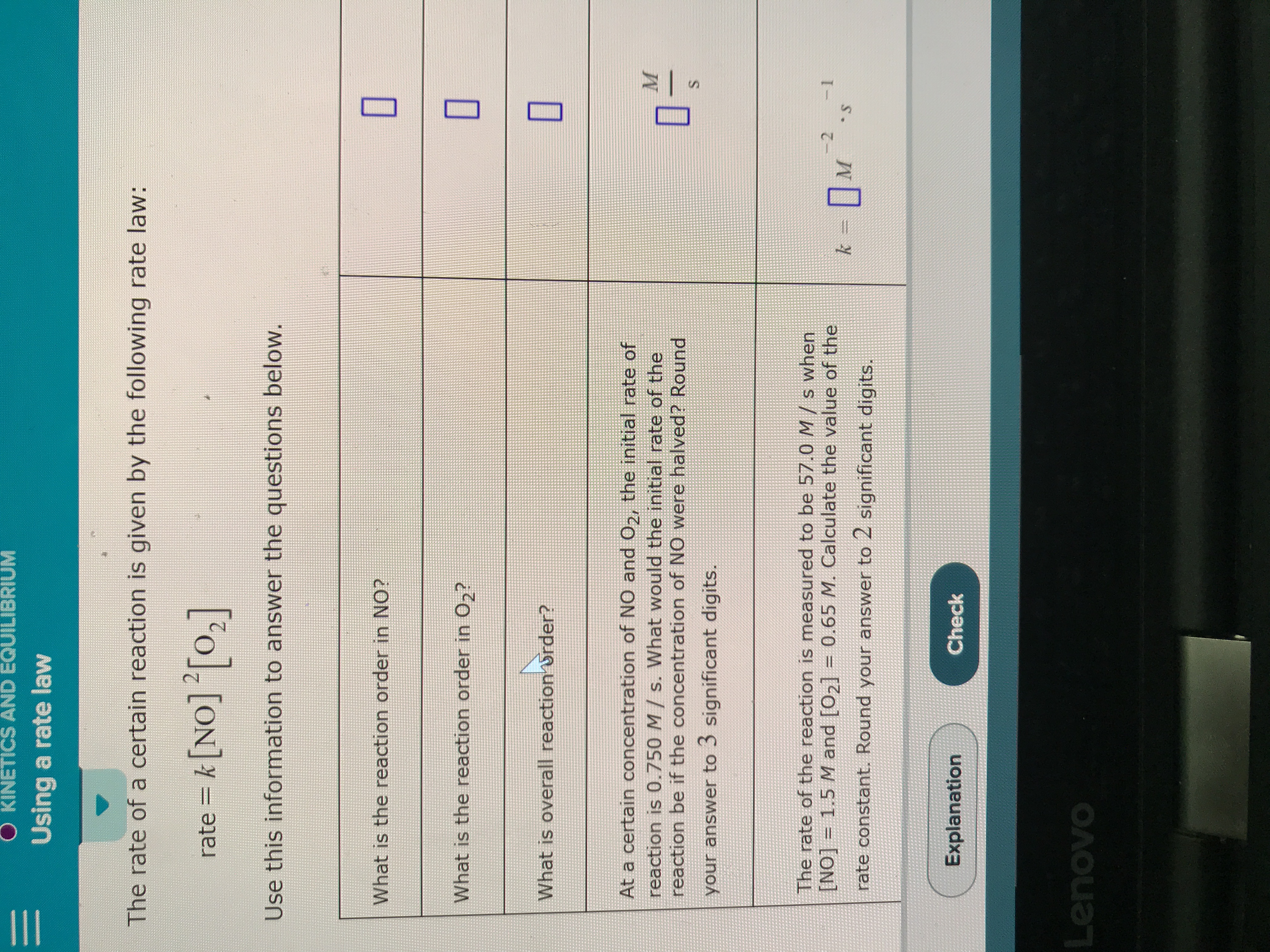# The rate of a certain reaction s given by the following rate law: rate = k[NO]2[O2]. (a) At a certain concentration of NO and O2, the initial rate of reaction is 0.750 M/s. What would the initial rate of the reaction be if the concentration of NO were halved? Round of your answer to 3 digits. (b) The rate of the reaction is measured to be 57.0 M/s when the [NO] = 1.5 M and [O2] = 0.65 M. Calculate the value of the rate constant.